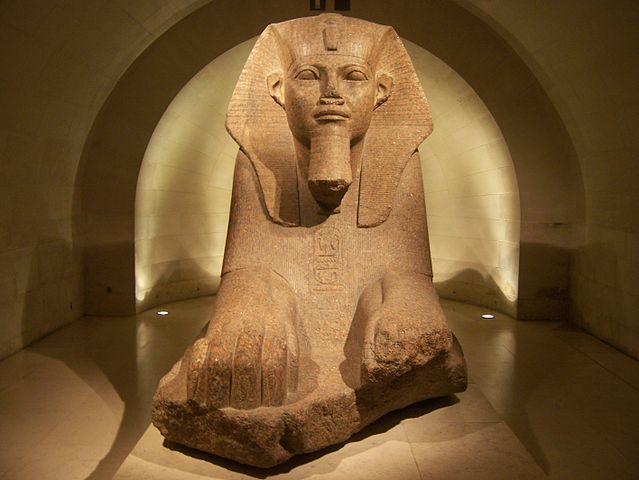Egyptian Floor Fractions

Algebra Level 5$x$ is a real number that satisfies $\dfrac{1}{\lfloor x \rfloor}=\dfrac{1}{\lfloor 2x \rfloor}+\dfrac{1}{\lfloor 3x \rfloor}+\dfrac{1}{\lfloor 5x \rfloor}$

Let the largest possible value of $x$ be $M$, and the smallest possible value of $x$ be $m$. If $M+m$ can be expressed as $\dfrac{p}{q}$ for positive coprime integers $p,q$, then what is $p+q$?

×